#### How to use Match function in Excel

The MATCH function allows you to determine the position of the search item in the range of cells. Excel is mainly used as a database to store large amounts of data, and frequently, you will have to search the Excel sheet for data.

As it is not at all practical to search the data manually, Excel offers a few functions to search the sheet for a specific value, like the MATCH function. The MATCH function returns the position of the searched value if successful, and returns the #N/A error value if the function is unsuccessful.

## How to use a MATCH function?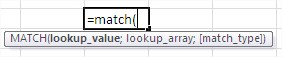The function syntax is as follows:

MATCH (lookup_value, range_lookup; [match_type])

• Lookup_value is an element we seek. This may be a number, text, logical value, or cell reference that contains one of these types of data.
• Range_lookup specifies the range of cells to be searched.
• Match_type is an optional argument (defaults to 1). It decides how to compare the values and looks for cells within the searched range. It may have the following values:

0 – the result is given as the first value in the field of equal sought,

1 – for sorted in ascending column gives the largest value less than or equal sought,

-1 – For sorted descending column gives the smallest value greater than or equal sought.

## Match function examples

Let us use the function in the following example: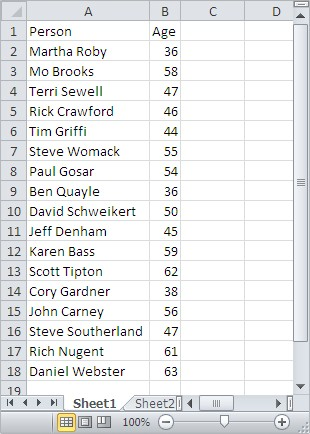To find a person who is X years old, enter the following formula:

= MATCH (X, B2:B18, 0)

Looking for a person at age 50, we obtain a result of 9 – and thus the ninth person on the list is 50 years old. Typing an analogous formula for a person who is 60 years old, we get an #N/A error. This means that the value was not found.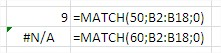To use a comparison of 1, sort the table columns by ascending age. To find the oldest person whose age does not exceed X years, enter the formula:

=MATCH (X, B2:B18, 1)

Since the table is a person aged 50 years, the function returns its position. In the case of finding a 60-year-old, get into the position of being 59 years old.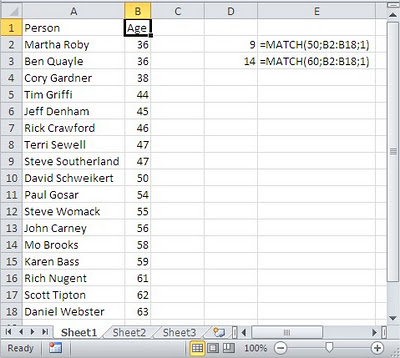Let’s practice with these examples. Open Excel and save your file as match.xlsx. Enter details as in the following image.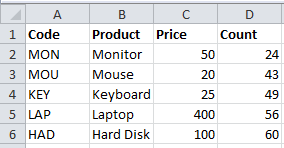Example 1: Click on cell F1 and enter the formula =MATCH(“MOU”,A2:A6,0).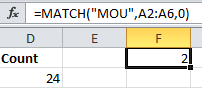Now the result is 2. The item MOU is in the second position in the array. We get the result as 2 because we started the array from A2 and the item is in the cell A3.

Example 2: Click on cell F2 and enter the formula =MATCH(“MOU”,A1:A6,0).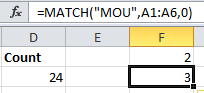Now the result is 3. The item MOU is in the third position in the array as we started the array from A1 this time.

Example 3: Click on cell F3 and enter the formula =MATCH(“Har*”,B2:B6, 0).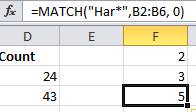Now the result is 5. Here, we use an asterisk to match any sequence of characters.

Example 4: Click on cell F4 and enter the formula =MATCH(“Har?”,B2:B6, 0). Now your screen will look like this: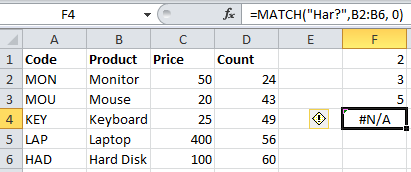Here, you get the result as #N/A. The question mark matches a single character and there is no item with a single character after Har so it returns #N/A.

Example 5: Click on cell F5 and enter the formula =MATCH(“LA?”,A2:A6, 0).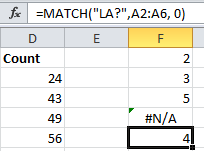Now the result is 4. Here, we use a question mark to match a single character.

Example 6: Click on cell F6 and enter the formula =MATCH(48,D2:D6, 1).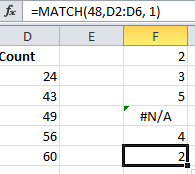Now the result is 2. Here, as the value of the type parameter is 1, the position of the largest value that is less than or equal to 48 is returned.

Example 7: Click on cell F7 and enter the formula =MATCH(62,D2:D6).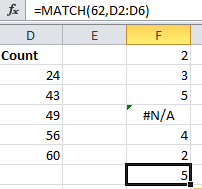Now the result is 5. Here, as the value of the type parameter is omitted, it has the default value of 1. Hence, the position of the largest value that is less than or equal to 62 is returned.

Example 8: Enter details in the A column as shown in the following image.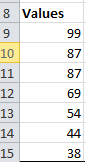Click on cell F8 and enter the formula =MATCH(68,A9:A15,-1).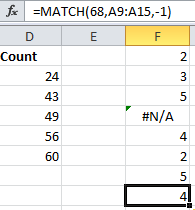Now the result is 4. Here, as the value of the type parameter is -1, the position of the smallest value that is greater than 68 is returned.

Example 9: Click on cell F9 and enter the formula =MATCH(43,A9:A15,-1).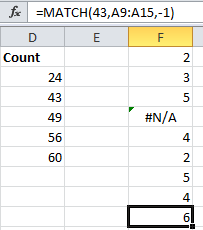Now the result is 6. Here, as the value of the type parameter is -1, the position of the smallest value that is greater than 43 is returned.

Example 10: Click on cell F10 and enter the formula =MATCH(87,A9:A15,0).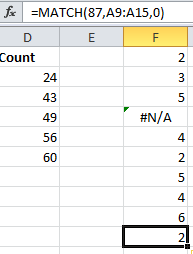Now the result is 2. Here, as 87 is repeated twice in the array, it returns the position of the first instance of 87.